# DAV Class 4 Maths Chapter 10 Worksheet 3 Solutions

The DAV Maths Book Class 4 Solutions and DAV Class 4 Maths Chapter 10 Worksheet 3 Solutions of Angles offer comprehensive answers to textbook questions.

## DAV Class 4 Maths Ch 10 WS 3 Solutions

Question 1.
Use the protractor and measure the following angles.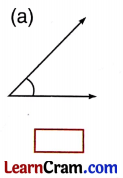45°70°110°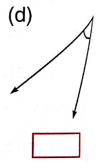35°90°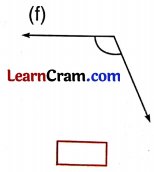115°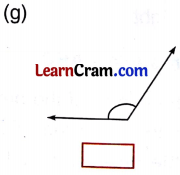120°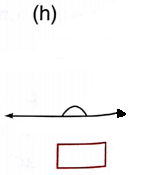180°Question 2.
Construct angles of the following measures. Label the angles and name them.
(a) 40°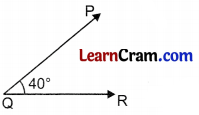∠PQR = 40°

(b) 75°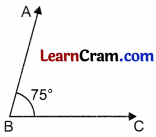∠ABC = 75°

(c) 90°∠XYZ = 90°

(d) 100°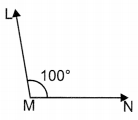∠LMN = 100°

(e) 120°∠DEF = 120°

(f) 155°∠STR = 155°

### DAV Class 4 Maths Chapter 10 Worksheet 3 Notes

Measuring Angles:
The standard unit of an angle is Degree. The symbol (°) is used for degree. We use protractor to measure an angle.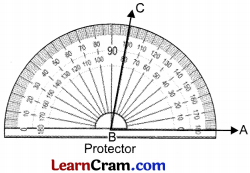There are two rows of numbers marked on the protractor. Numbers are marked from 0 to 180. It has two scales in reverse order. Measuring ZABC

• Step I: Place the centre of the protractor on the vertex B of ∠CBA. Adjust the arm BA.
• Step II: Look for the scale which begins with zero.
• Step III: Read the mark on the protractor where the other arm ($$\overrightarrow{\mathrm{BC}}$$) of the angle crosses the scale on the protractor.

∠ABC = 80° as shown in figure. Angles opening to left increases from left to right. Angles opening to right increases from right to left.